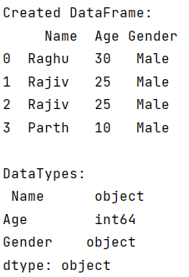# How to check the dtype of a column in Python Pandas?

Given a Pandas DataFrame, we have to check the dtype of a column. By Pranit Sharma Last updated : September 22, 2023

Pandas is a special tool that allows us to perform complex manipulations of data effectively and efficiently. Inside pandas, we mostly deal with a dataset in the form of DataFrame. DataFrames are 2-dimensional data structures in pandas. DataFrames consist of rows, columns, and the data. The Data inside the DataFrame can be of any type.

## Problem statement

Given a Pandas DataFrame, we have to check the dtype of a column.

## Checking the dtype of a column in Python Pandas

For this purpose, we will use pandas.DataFrame.dtypes attribute which returns a series with the data type of each column. We have to just use .dtypes along with the DataFrame's object. Consider the below-given code statement for this,

```# Returning the dtype of all columns
result = df.dtypes
```
Note

To work with pandas, we need to import pandas package first, below is the syntax:

```import pandas as pd
```

Let us understand with the help of an example,

## Python program to check the dtype of a column in Pandas

```# Importing pandas package
import pandas as pd

# Creating a dictionary
d = {
'Name':['Raghu','Rajiv','Rajiv','Parth'],
'Age':[30,25,25,10],
'Gender':['Male','Male','Male','Male']
}

# Creating a DataFrame
df = pd.DataFrame(d)

# Display DataFrame
print("Created DataFrame:\n",df,"\n")

# Returning the dtype of all columns
result = df.dtypes

# Display result
print("DataTypes:\n",result)
```

### Output

The output of the above program is: